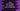# Java program to find the sum of digits of a number until a single digit is found## Java program to find the sum of digits of a number until a single digit is found:

In this Java programming tutorial, we will learn how to find the sum of all digits of a number, until a single digit is found.

For example, for the number 345, the sum of its digits is 3 + 4 + 5 i.e. 12. But, 12 is greater than 10. So, we have to find the sum of digits again, i.e. 1 + 2, which is 3 and this is a single-digit number. So, it will print 3 for 345.

This program will take one number as input from the user and it will print the single digit value.

### Algorithm to follow:

We will follow the following algorithm:

• Take the number as input from the user.
• Find the sum of the digits of the number.
• If it is not a one digit value, find its sum of digits again.
• Keep doing it until you get asingle-digitt value.
• Print that number.

### Java program:

Let’s write down the complete Java program:

``````import java.util.Scanner;

public class Main {
private static int findSumOfDigits(int no) {
int sum = 0;

while (no > 0) {
sum += no % 10;
no /= 10;
}

return sum;
}

public static void main(String[] args) {
int no, sum;
Scanner sc = new Scanner(System.in);

System.out.println("Enter the number: ");
no = sc.nextInt();

sum = no;

while (sum > 9) {
sum = findSumOfDigits(sum);
}

System.out.println("Sum for " + no + " is: " + sum);
}
}``````

In this program,

• no and sum are two integer variables to hold the user input number and the sum of digits.
• sc is a Scanner object to read the user inputs.
• It asks the user to enter a number. It reads the number by using the Scanner object and stores that in the no variable.
• We are assigning the value of no to sum.
• The while loop will run until the value of sum is more than 9.

• Inside the loop, we are finding the sum of the digits of sum by using the findSumOfDigits method and assigning it to sum.
• This loop will stop once we get a single-digit value of the sum.
• findSumOfDigits method takes a number and returns the sum of the digits of that number.

• It initializes a sum variable as 0 and by using a while loop, it finds the sum of the digits.
• At the end of the program, we are printing the single-digit sum.

### Method 2: O(1) solution:

There is another easy way to solve this problem. If a number is divisible by 9, then the single-digit sum we found by adding the digits of the number is always 9.

For example, 8802 is divisible by 9. So, if we add the digits, it will be 8 + 8 + 0 + 2 = 18, and if we add the digits of 18, it will be 9. Only for 0, it will be 0.

For all numbers, we can either represent it as 9 * n or 9 * n + d. For all 9 * n numbers, it will be 9 and for 9 * n + d, it will be d.

Let’s write down the program with this algorithm:

``````import java.util.Scanner;

public class Main {
private static int findSumOfDigits(int no) {
if (no == 0) {
return 0;
} else if (no % 9 == 0) {
return 9;
} else {
return no % 9;
}
}

public static void main(String[] args) {
int no;
Scanner sc = new Scanner(System.in);

System.out.println("Enter the number: ");
no = sc.nextInt();

System.out.println("Sum for " + no + " is: " + findSumOfDigits(no));
}
}``````

So,

• We changed the findSumOfDigits method.
• It takes one number as the parameter

• Returns 0 if the number is 0
• Returns 9 if the number is divisible by 9.
• Else, it returns no % 9, or the remainder if we divide no by 9

If you run this program, it will print output as like below:

``````Enter the number:
8802
Sum for 8802 is: 9``````

We can also use ternary operators to write it in just one line:

``````import java.util.Scanner;

public class Main {
private static int findSumOfDigits(int no) {
return no == 0 ? 0 : (no % 9 == 0 ? 9 : no % 9);
}

public static void main(String[] args) {
int no;
Scanner sc = new Scanner(System.in);

System.out.println("Enter the number: ");
no = sc.nextInt();

System.out.println("Sum for " + no + " is: " + findSumOfDigits(no));
}
}``````

It will print a similar result.